## How To Find A Reference Angle In Calculus

We'll discuss how to find a reference angle and discuss some of the basics of trigonometry, just in case it’s been a while since you’ve taken trig.

Trigonometry plays an important role in preparing you for calculus. Learning the right concepts of trig, and understanding them well can help you succeed in calculus. Learn more about how to 𝛳find a reference angle.

Whether you’re enrolled in a trigonometry or calculus course, both studies have an important relationship. Trigonometry, like algebra, is a common class to take before you move on to calculus. While you might have great success in calculus without experience in trig, it can help you understand many difficult concepts more easily.

In this article, we will discuss how to find a reference angle and discuss some of the basics of trigonometry, just in case it’s been a while since you’ve taken trig or you’re unfamiliar with some of the concepts you learn in trigonometry.

## A Quick Look At Trigonometry

Before we can show you how to find a reference angle, you should understand the basics of angles and where they appear on a graph. Trigonometry is the study of angles and how they are used as a means of indirect measurement, especially when distance can be difficult to measure directly.

While trigonometry can often be an overwhelming course in mathematics, there are several opportunities to use the skills you learn in real life applications. Surveyors, engineers, and even architects use angles to measure distances and ensure that measurements are accurate.

Let’s take a quick look at angles and brush up on some of the basics. A ray, which is a line that has a defined endpoint also extends endlessly in one direction. The endpoint of the ray is placed at the origin of an x and y-axis system; the ray lies on the positive x-axis, but you can rotate the ray.

When using the x and y-axis system, rotations that go counterclockwise are positive while clockwise rotations are negative.

If you’re already familiar with angles and graphing, you might remember that an x and y-axis system is divided into four quadrants on the Cartesian plane, which are simply numbered one through 4. The location of the quadrants are as follows:

Quadrant one is located at the top right, and both x and y points are positive. Moving in a counterclockwise rotation, quadrants 2, 3, and 4 continue (with 4 located beneath quadrant one with negative points on the y-axis and positive points on x-axis).

The angle is measured on the ray’s starting position, which is along the positive x-axis (also known as the initial side) and ending at its terminal side.

Before we move on to discussing reference angles, let’s do a little refresher on the types of angles:

• A straight angle is 180°
• A right angle is 90°
• Angles between 90 and 180° are known as obtuse
• Angles between 0 and 90° are acute

There are many other aspects to explore in Trigonometry, but it is most important to discuss things like quadrants as they play an integral role in finding a reference angle.

## Finding A Reference Angle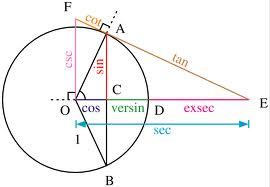IMAGE BY: flickr

Before we tell you how to find a reference angle, it’s important to define what a reference angle is first. A reference angle is a positive acute angle, which as we just mentioned above means that is between 0 and 90°; a reference angle is never any other type of angle.

Reference angles are found inside of the reference triangle and close to the origin, which is also the vertex. The positive acute angle is between the x-axis and the terminal side.

Before we discuss each quadrant, it’s important to remember that they are ordered from one to four in a counterclockwise direction. You can still find the reference angle is you rotate negatively or if your angles are more than 360° (in our examples we will only find the reference angles for degrees between 0 and 360.

To help you find the reference angle more easily, it’s important to keep the following information on hand. As you practice with more examples, you may not need to reference this table. We will look at each quadrant, the measure of the angle theta (which is used to represent an angle) or 𝛳, and the measure of the reference angle.

Quadrant I has a measure of angle theta is 0° to 90° and the measure of reference angle (R) is theta (𝛳) or R=𝛳.

Quadrant II has a measure of angle theta is 90° to 180°, and the measure of reference angle is 180° minus 𝛳 or R=180°-𝛳.

Quadrant III has a measure of angle theta is 180° to 270°, and the measure of reference angle is 𝛳 minus 180° or R=𝛳-180°.

Quadrant IV has a measure of angle theta is 270° to 360° and the measure of reference angle is 360° minus 𝛳 or R=360°-𝛳.

Considering the information we gave you for each quadrant, it’s time to take a look at some examples and try to find the reference angle when we know what 𝛳 equals.

To find the reference angle, sketch out the angle on a graph. Drop a perpendicular to the x-axis to form a triangle.

### Example 1:

If 𝛳=135°. You can start by graphing your picture, and you will notice that 135° is in Q II. To find out the reference angle, simply use the quadrant II formula from above. 180-135=45. The reference angle, when 𝛳=135°, is 45°. This is correct because it’s acute and positive.

Remember, the reference angle can never be negative or more than 90°. If your answer is negative more than 90°, you need to look at your problem and start over.

### Example 2:

If 𝛳=32°. 32° is in Q I. To find out the reference angle, simply use the quadrant I formula from above. Q I is easy as 𝛳=𝛳. The reference angle of 32° remains unchanged and is 32°.

### Example 3:

If 𝛳=329°, 329° is in Q IV. Looking at the formula, 360-329=31. The reference angle, when 𝛳=329°, is 31°.

### Example 4:

If 𝛳=251°, 251° is in Q III. Use the quadrant III formula from above to find the reference angle, 251-180=71. The reference angle, when 𝛳=251°, is 71°.

### Example 5:

In our next example, we take a look at negative thetas. Since we know that a reference angle must be positive, it may be confusing to put a negative theta in the formula. One of the key differences between negative and positive thetas is that you should rotate clockwise rather than counterclockwise when searching for the correct quadrant.

Remember, your reference angle will not be negative. You should only use the negative number to help you determine which quadrant you’re in.

If 𝛳=-150°, -150° is in Q III. It’s important to note that even though you the negative theta is in Q III, you cannot use the formula as your result will be negative. When finding the reference angle, you will treat the theta as if it is positive. The formula you will use will be Q II (rather than Q III).

So, 180-150=30. The reference angle, when 𝛳=-150°, is 30°.

### Example 6:

Let’s try finding the reference angle using another negative theta. If 𝛳=-20°, -20° is in Q IV. When we look at the Q IV formula, it’s 360°- 𝛳. If we followed the formula as is, we would still end up with a negative reference angle. So ignoring the negative sign, 𝛳=20°, which puts it in Q I and the reference angle is 20° since 𝛳=𝛳.

### Example 7:

At times, you may be asked to find the reference angle for thetas that are more than 360°. Let’s find the reference angle when the theta is 960°. A 960° angle is the equivalent to a 240° angle. You can find this out by subtracting 360° from 960° two times.

Since 𝛳=240°, you will use the formula from Q III. 240-180=60. The reference angle, when 𝛳=960°, is 60°.

## Final ThoughtsIMAGE BY: flickr

Finding the reference angle is often considered as the “fast track” to finding the angle of a triangle. We gave you the basics of the reference angle, but as you progress in your math course, you’re likely to use the reference angle to find the sine, cosine, and tangent of the non-acute angles.

Like all new material you learn in a math course, memorizing can be helpful, but it may be more beneficial to practice until you can find the reference angle without referring to the quadrant formula table.

As with any new concept, you can expect some problems to be easier than others. Allow yourself to try out all kinds of reference angle examples, which include negative numbers and over 360°.

The video may take a few seconds to load.

Having trouble Viewing Video content? Some browsers do not support this version – Try a different browser.## Problem 14: Power Rule for Integration

Looking to figure out how to sole the power rule for integration for your calculus homework? We have the answers at calculus-help.com.

2019-2020

Find the antiderivatives of each with respect to x, without using u-substitution (i.e., without changing variables):

(a)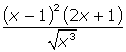(b)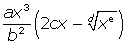, assuming a, b, c, d, and e are constants

## Integral Power Rule Solution:

(a) For the integral power rule solution, start by expanding the numerator, squaring (x – 1) and mutliplying by (2x + 1). Rewrite the denominator as a term with a negative exponent, and then distribute it through the quantity.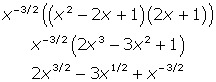Now you can antidifferentiate by adding 1 to each power individually and multiplying the coefficient by the reciprocal of the resulting exponent. In other words, for the first term, add 1 to 3/2 to get 5/2. Then, multiply 2 by the reciprocal of 5/2, which is 2/5.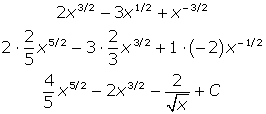Don’t forget that a general antiderivative (i.e., an indefinite integral) must always contain “+ C.”

(b) Follow the same procedure, beginning by writing the radical term with a fractional exponent. Then,  distribute and find the antiderivtaive. The variables make it marginally more difficult, especially when you add 1 to the weird variable powers.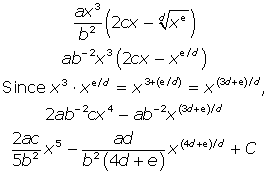To apply the power rule for integration to this type of function, you have to remember an important rule from algebra.

If we can write the function using exponents then we most likely can apply the power rule.

Let’s solve this problem:

∫ √x+4 dx

Before even using any calculus, you can rewrite the function using the above rule with exponents. So, you have exponents and can apply the power  rule.

It becomes:

∫√x + 4 dx = ∫x^1/2 +4 dx

Then, you can apply the power rule.

It then becomes:

x^1/2 +4 dx= (x^(1/2+1)) / ( 1/2 + 1) + 4x + C

When simplified, you get the final answer.

That is:

(x^3/2 / 3/2) + 4x + C

Which equals:

(2/3 * x^2/3) + 4x + C

The final answer can usually be written with exponents, like we did here. Or, it can be written with roots. However, your teacher or professor may have a preference, so make sure you ask!

## Finding the Power Rule for Integration

As with anything else related to calculus, perfecting the power rule for integration will take a lot of practice if it doesn’t come to naturally.

Make sure you look up practice problems online! You can browse our other calculus problems here. Best of luck!

The video may take a few seconds to load.Having trouble Viewing Video content? Some browsers do not support this version – Try a different browser.##2019-2020 Derivative Table

Two functions, f(x) and g(x), are continuous and differentiable for all real numbers. Some values of the functions and their derivatives are given in the following table.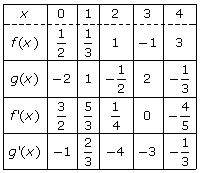Based on that glorious table, calculate the following:

(a)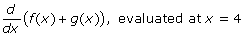(b)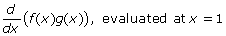(c)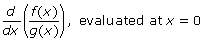(d)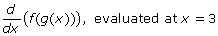### Solution:

(a) Take the derivative of each function separately (the derivative of a sum is equal to sum of its derivatives) and plug in 4 to each to get your answer. Reference the chart for the values of f ‘(4) andg(4).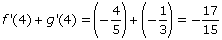(b) This time you have to use the Product Rule, because f(x) and g(x) are multiplied. Once again, after you apply the derivative rule, just nab the needed function and derivative values from the chart.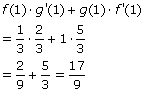(c) This time it’s the Quotient Rule that has to be applied.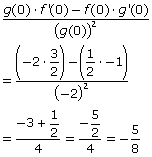(d) How about a big, warm welcome for the Chain Rule! Remember, you apply the Chain Rule when one function is composed with (inside of) another. To differentiate, take the derivative of the outer functionf(x) while leaving g(x) alone inside f(x). Then multiply by the derivative of g(x).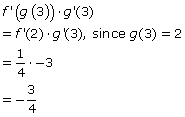The video may take a few seconds to load.Having trouble Viewing Video content? Some browsers do not support this version – Try a different browser.## Rectangle Calc: Find A – Solving the Problem

Trying to solve the area of a rectangle? Can't quite remember how it'sdone? Check out this simple formula to solve rectangle calc: find A.

Rectangle Calc: Find A

When you’re facing a rectangle calc: find A problem, there’s a simple formula to remember. Follow the steps below, and you’ll have your answer in no time.

## What Does A Stand For?Photo by Louis Bauer from Pexels

Before diving into the answer to your math problem, there are some things you need to know first. You know that you are dealing with a rectangle, a familiar shape, and the elements of a rectangle. There are four sides with two different lengths, space inside of the box this shape creates, and a few factors to the problem you’re viewing as well.

Knowing more about these factors will help you solve A. The longer lines comprising the rectangle are the length lines. Meanwhile, the shorter sides are the width. These are often denoted as X or L for length and Y or W for width.

Keep in mind that these can be any letters in the alphabet. It doesn’t matter which letters they are when solving the
problem. All you need to do is identify which is the length and the width for this rectangle calculation.

Both of these numbers are used to find A, which stands for area. The area of a shape is a surface measurement, identifying the entire area taken up by the side of the shape you’re looking at. In this case, the area for your 2-D rectangle is the entire space contained inside its four walls.

The equation to find an area is straightforward, with one main variation. Once you know the equation, solving it is a piece of cake.

It looks like this:

A = X • Y

By multiplying the length (X) by the width (Y), you can quickly determine the area within any rectangle. You can also use this equation on squares, but other shapes require a different setup since they have varying numbers of sides.

## ​Why Do I Need This?Image by pexels

Not a lot of people are motivated to solve a math problem “just because,” which is why it helps to visualize a real-world instance where an equation is used. You might wonder if there ever is a time when you might need to solve for A, but the truth is that people have been doing so for thousands of years.

Ancient Mesopotamian writings first talked about finding the area inside of a space. This civilization used the same equation for farming, determining how large a plot of crops would be. They knew if one farm was twice as wide and three times as long as another, the larger farm would be six times the size.

Around 2,500 B.C., architects were using area equations for two-dimensional triangles to construct the pyramids at Giza. Chinese cultures were working on advanced area equations for multi-sided shapes by 100 B.C.

The orbits of planets in our solar system were calculated using this equation, too. Johannes Keppler was able to measure sections of their orbits in the early 1600s, calculating the area of an oval to determine their path around the sun.

Sir Isaac Newton expanded on this concept, using area equations to develop the mathematical field of calculus. So, ancient humans and those in the relatively not-so-distant past relied on this formula for everything from daily life to advancing discoveries.

You can use this equation in your everyday life, as well. If you were buying carpet or flooring for a room in your house, you would need to know the area to buy the correct amount of materials. People who knit and sew need to know the area of a quilt before making it. The same goes for wallpaper and painting.

How much area does one bucket of paint cover? Will two be enough for your bedroom, or will you risk wasting your money on buying more than you need? Another real-life example would be construction work, where equations for shapes are continuously used to design and build different projects.

## ​How to Solve for A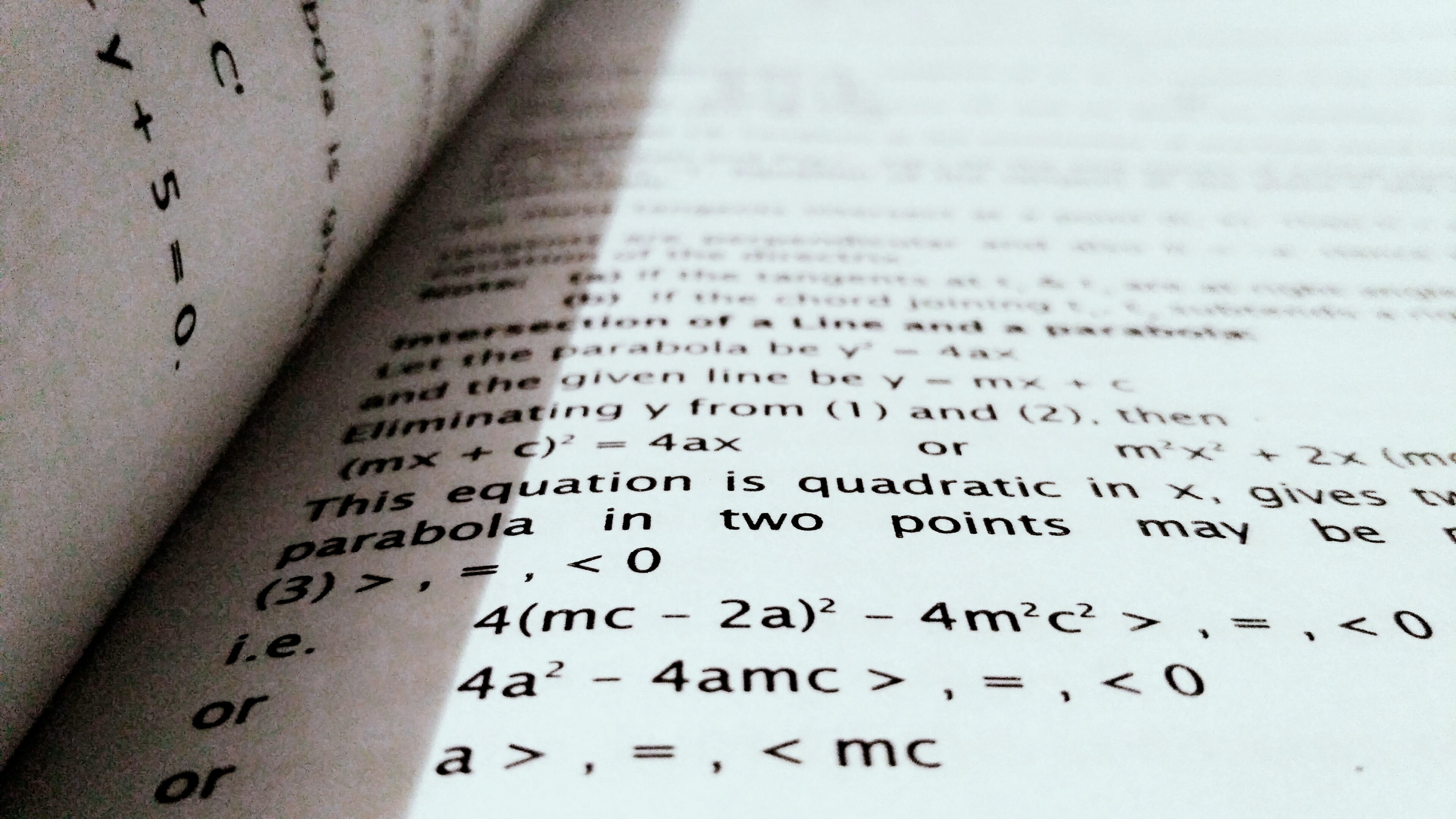Photo by Deepak Gautam from Pexels

Solving for A is a simple process. Start with the equation from earlier, A = X • Y, then plug in the numbers for length and width. The majority of math problems will give you this information until you head into more complicated levels of calculus.

If your rectangle had a length of 15 and a width of 8, your equation would become A = 15 • 8. From there, all you have to do is multiply the two to get your answer. So:

A = 15 • 8

A = 120

While 120 is your numerical answer, you’re not finished with this problem just yet. Each rectangle you work within these types of calculations will have a specific measurement. This could be anything from inches to yards and beyond. To finish your answer, add in the unit of measurement after the number.

If this problem were in feet, A = 120 ft would sound like the correct answer. However, the area of a rectangle is measured in square units. So, the answer is 120 square feet. You can abbreviate this to 120 Sq — Ft. or 120 in2 to save time.

Having the unit next to the number is essential when dealing with real-life scenarios. While you might not be handling those now, it’s an excellent idea to get in the practice of including the form of measurement.

This formula works for any numbers you plug in, too. If A = 7 in • 5 in, then the area is 35 in. If A = 364 yds • 196 yds, then A = 71,344 yds. Now that you know how to solve for area, you can handle any “rectangle calc: find A” problem.

Keep practicing honing your skill and speed with this equation. The more you practice, the easier these math problems become. Can you find A when the length of a rectangle is 12 in, and the width is 11 in? What about 20 ft and 9 ft?

## ​Upping the AntePhoto by Kaboompics .com from Pexels

Of course, you won’t always run into easier problems with whole numbers. The length and width can include fractions, and often does in real-life scenarios. These can be represented by a number like 11.25 or a mixed number such as 115/8. Solving for these is a little more complicated, but not as challenging as you might think.

Look at the original problem above, A = 15 • 8. Suppose those numbers changed, making the length 155/8 and the width 81/2. Both of those measurements are in feet this time. Do you think you can still solve it? The equation now looks like this:

A = 15 • 8

To solve this problem, you will need to follow the same area formula while using your knowledge about multiplying fractions. The next step would be to change the length and width from mixed numbers to improper fractions.

15 =   =    and   8 = =

Next, you can apply the multiplication formula for fractions to combine your length and width.

Then, you need to simplify the answer from an improper fraction back to a mixed number. Finally, add in the unit of measurement to complete your answer to the problem.

A = 132 ft2

Do you see? Solving a more complex equation only takes a little extra time now that you know the formula for area. If all of that seemed a little tricky, don’t worry. Keep practicing, and you’ll get the hang of it in no time. Soon, you’ll find yourself solving “rectangle calc: find A” problems in a matter of minutes.

## ​With Only One ElementPhoto by rawpixel.com from Pexels

You will eventually run into problems where neither the length or width is known. In this case, the diagonal is usually given for you to use the second area formula for a rectangle. This formula is:

A = d2 ÷ 2

If the diagonal length, from one end corner to another, was 13 inches, then what would the answer be? Here’s the solution:

A = 132 ÷ 2

A = 169 ÷ 2

A = 84.5 in2

### A Note on SquaresImage by pexels

With squares, you have a shape that is even on all sides. This allows you to further simplify the formula to save time. While you’ll work more with rectangles, any time-saving tricks in math are worth your while.

You can stick with the same A = X • Y formula if you want or simplify that to A = s2. S stands for the length of any one side, which is then squared to find the area. So, the answer A = 122in is A = 144 square inches.

## ​Conclusion

Finding the area of a rectangle is simple once you know which formula to use. Since there are only two, you can master this mathematical concept with ease. Keep practicing to improve your skill and speed at solving these problems!

The video may take a few seconds to load.Having trouble Viewing Video content? Some browsers do not support this version – Try a different browser.## What is Multivariate Normal Distribution in Calculus?

In statistics, there are three-dimensional functions called multivariate systems, one of them is the multivariate normal distribution.

## What is Multivariate Normal Distribution in Calculus?

Normally, when you’re working in calculus and statistics, you’re dealing with a two-dimensional curve. You have a simple graph with points plotted on x and y coordinates. Functions are expressed in terms of x and y because you’re only working with two dimensions. Of course, in the real world (i.e., practical applications, you aren’t likely working in two dimensions.

Therefore, you have to have an addition. You’re working on the z-axis as well as the x- and y-axis. On a graph, this is plotted out by using another axis protruding perpendicularly to the other two, and while it can look confusing, you get used to it over time.

Multivariate functions, also called multivariable functions, show up in statistics and calculus primarily. You won’t see them much in something like trigonometry, but you’ll see them in various scientific fields. The most common place you’ll find these functions is in physics when calculating vectors. Vectors are lines or patterns of force that operate in three-dimensional space.

## What is the Normal Distribution?

Before going into depth about what the multivariate normal distribution is, let’s talk about the univariate distribution.

In probability theory, which underlies a lot of statistics, you often hear about something called the bell curve. The bell curve is the most basic form and arguably the most important concept in all of statistics, calculus, and algebra.

Normal distribution of data simply means that, for any given set of data points, most of them are going to cluster around the median point, with fewer points existing at the upper and lower bounds of the function.

In other words, if you have a group of people categorized by various heights, more people’s height measurements are going to tend toward the average height of the sample You’ll only have a few outliers, In statistics, the term ‘outlier’ refers to any point of data that lies on one of the ends of the bell curve. In the example, it would refer to a very tall or very short person.

Several important measurements in statistics include:

• Mean

• Median

• Mode

• Standard Deviation

The mean is more commonly referred to as the average, and it’s where most of your data will converge on any graph. To find the mean of a set of data, you take the sum of all the data values and divide by the number of values. If you had five data points with a total of 350, the mean would be 70. On a graph, you may not be able to plot this.

The median is the middle of the set of data. When arranged from lowest to highest, the median is the middle number if there is an odd number of data points. Otherwise, you would take the mean of the two middle values to get the median. This is also not easy to spot on a graph unless you have a discrete, bounded function when you would likely take an integral.

The mode is the point of data that occurs the most in a given sample. When plotted as a function, you’ll see it as a horizontal line segment.

The standard deviation, meanwhile, dictates the overall spread of the data. A sharper bell curve indicates that more data clusters near the median, while a smooth curve shows that data exists on a more even distribution across the x-axis.

Generally, the bell curve has a predictable percentage: Over 68 percent of data points will fall within 1 standard deviation of the mean. 95 percent of data will fall within 2 standard deviations, and up to 99.7 percent within 3 standard deviations.

## Three-Dimensional Graphs

At first, it can seem difficult to graph and read graphs in three dimensions because you’re working on a two-dimensional sheet of paper or screen, but it gets easier. All you’re doing is adding an axis, and shifting the relative positions around. The x-axis, which holds the independent variable in many functions, runs “forward” and “backward” into the page.

The y-axis runs vertically as always. Meanwhile, the z-axis takes the former place of the x-axis. X and z can sometimes switch places, depending on the program you’re using or what is easier to read. If you have the liberty to do so, it may make more sense to have z be the new axis pointing out of the page.

Points on a three-dimensional graph are expressed as (x, y, z) just as they are in two-dimensional ones. A big difference is what happens when you have a single-variable constant like x = 2. Instead of a line, you now have to think of the solution as a plane.

For any function or system expressed in terms of f(x), you have to think of it as a line, curve, or enclosed shape. When you add the third dimension, it’s a solid form, or a set of points encompassed by a given plane passing through intercept points. To find intercepts, you set each variable in turn to zero and solve the equation.

### Vectors

To understand what multivariate normal distribution is, you have to understand vectors. We mentioned vectors earlier as lines of force. This is the purely physics-oriented definition. In math, a vector has two properties: its magnitude and its direction. The magnitude of a vector on a graph is represented by the length of a line segment, starting from the origin point.

The direction of a vector is indicated by a line drawn from the origin point to a point given by the form R(t) = <f(t), g(t), h(t)>. Each of the components is a function on its own.

Therefore, you can easily determine a vector by taking its parts as a system. In calculus, you might have to express the vector in terms of time, which is why it’s written using the variable t as a function of each dimension. For example, if you’re graphing a curve, you might need to find out what the curve looks like between values of t = 1 and 3.

Vectors are often a function of time and force. In other words, you want to know how a force changes over time both in magnitude and direction. This is why you often see multivariate graphs of advanced functions. For example, you might want to know how much force an engine is exerting on a car to make it travel, and to find how efficient the engine is with the driver’s input.

Vectors are expressed in both positive and negative terms when referring to the direction of a force. If the positive direction is right, then a vector moving left is negative. Unless you get into some highly advanced theoretical physics, you’re not going to see a negative variable for time.

### Multivariate Normal DistributionImage source: Unsplash

Multivariate normal distribution takes the standard bell curve and extrapolates it over three dimensions. In other words, if you were to look at a three-dimensional graph of the function, it would look like an upside-down bowl. This works by treating y and z as the same variable.

The multivariate distribution is a generalized extrapolation of the bell curve, and the bell curve itself may not hold for all sets of data.  Any data set where there is an outside influencing factor on results will have a skewed normal distribution. However, in cases with a completely random data set, you can expect to see a bell curve.

A multivariate distribution exists when you have two random variables. Although in theory, you can have as many as you want when it comes to physically graphing the functions we cannot create graphs in higher than 3-dimensional space. Therefore, for practical purposes, you’ll only see functions using three variables on graphs.

### Practical Uses of Multivariate Functions

Since we’re getting into these branches of higher mathematics, you might have the same question many students do: what’s the point of learning it all? Aside from passing the next exam, learning calculus and statistics may not seem practical. However, if you’re in the business or scientific spheres, this knowledge can come in handy.

Using multivariate functions, including the multivariate normal distribution in calculus, can help you visualize or calculate the effects of multiple variables at once. Many factors in practical applications, like earth sciences or the previously-mentioned efficiency of a car engine at different speeds and times, require multivariate functions to graph effectively.

## Final ThoughtsImage source: Unsplash

Multivariate normal distribution in calculus is a field that carries over from statistics and trigonometry. It’s a good way to extrapolate data, but only if the data is completely randomized. Functionally, this type of function is an extension of a two-dimensional idea into a three-dimensional function.

The best way to graph these functions is to draw a box and mark it off in grid spaces. However, most of the time the numbers will be too complex – as in, unpleasant-looking fractions – to graph neatly. You’ll need a computer or calculator to provide you with the points you need.

The video may take a few seconds to load.

Having trouble Viewing Video content? Some browsers do not support this version – Try a different browser.## Rectangle Calc: Find P – The Concepts and Explanations

Having trouble solving a rectangle perimeter equation? No worries! Thisguide is here to help you tackle even the toughest rectangle calc: find p.

Rectangle Calc: Find P

So, you’re stuck on a math problem and can’t figure out how to solve for P. You know P has to equal something, and you think you remember how the equation for this problem goes, but you’re unsure.

Don’t get frustrated. You’ve found the answer!

Whether you’re taking this level of math for the first time, working on a project that requires a little computing, or just trying to help someone out with their homework, this article is here to walk you through any rectangle calc: find P problem in existence.

With a quick refresher and a few easy steps, you’ll be tearing through your problem in no time flat. Here’s everything you need to know about solving for P.

## ​What Does the Letter P Stand For?Photo by Startup Stock Photos from Pexels

Before attempting to tackle any equation, it helps to know what you’re looking at. In this case, you’re dealing with a rectangle. Simple enough, right? You’ve known this shape since you were a young child. What you need to know are the dimensions to this rectangle.

Your math problem also has letters on the inside or outside of the rectangle. These are most often X and Y. The letter X is located on a more extended section of the rectangle, while Y is found on a shorter part. These represent the length and width of the shape’s sides.

Keep in mind that their positions could be reversed, or they could be different letters entirely. It doesn’t matter which letters they are, all you need to know is that they represent the two different lengths of lines used to form the rectangle.

Across the shape from X is a line of the same length, which is true for Y as well. The longer lines are always going to be the length of the rectangle while the shorter two represent the width. If it helps you visually, you might want to write in L an W for these measurements.

Chances are, the letter P isn’t anywhere to be found on your rectangle. Instead, it’s in the equation for this math problem. P stands for perimeter, which is the sum of all the lines that create a shape. For any rectangle, that means it the sum of both width and length lines combined.

The way an equation looks in a “rectangle calc: find P” problem has three variations. It can look like either:

### Formula Derivation

P = x + x + y + y

P = 2x + 2y

or

P = 2(x + y)

All three of these equations produce the same answer to any problem, so use the one you are most comfortable with. Most math courses with use the third variation, P = 2(x +y). Getting familiar with this form of the equation will save you a lot of time as you progress to advanced problems and higher levels of mathematics.

## ​Why Solve for the Perimeter?Photo by Startup Stock Photos from Pexels

Aside from simply passing a course, you might wonder why anyone would need to solve for P in the first place. Is there a practical, real-life situation where this problem occurs? If so, when and how? Your textbook might give you a single example, but that isn’t the whole picture.

You might be surprised to learn that this equation is used relatively often, most notably in construction as well as some home uses. Imagine that you want to put a fence around your yard to keep your dog inside, for instance.

Fencing isn’t purchased in feet; it’s bought by area. You need to know the area of your yard to buy the right amount, which requires you to know the perimeter first. If you have someone put the fence in for you, they would have to do the same thing.

Architects drawing up designs for a house and construction workers must solve for P when creating a foundation. County codes and laws dictate how far away one home must be from another, which means a perimeter needs to be found to stay compliant when building.

If a farmer builds a barn to house their horses, knowing the perimeter of the barn itself and each stall allows them to maximize space while minimizing the cost of construction materials. The same is true for any construction project.

Companies that install swimming pools also have to solve for P. Their goal is to maximize the area around the pool within a person’s yard. A perimeter is used for the yard, the pool itself, and the surrounding area.

A less complex task that you do every year is gift wrapping. While you might not think about it, deciding on the amount of wrapping paper needed for a box uses the same formula. You can always eye it up, but solving for P would allow you to create a neatly wrapped gift with the least amount wrapping paper.

These are just a few examples of where perimeter equations are used in real life. The list goes on and on, making this a valuable piece of information for a wide variety of careers and home projects.

## ​How to Solve for PImage by pexels

When you receive a “rectangle calc: find P” problem, the first thing to do is identify your length and width. You only need to measure one long side and one short side of the rectangle since they are equal in length to their parallel sides. In most math problems, these numbers are given to you.

Let’s say the length of a rectangle was 7, and the width was 4. That would mean that X or L = 7 and Y or W = 4. You can rewrite any of the equations from earlier in this article to reflect these new values.

P = 7 + 7 + 4 + 4

P = 2(7) + 2(4)

or

P = 2(7 + 4)

Solving any of those variations of the perimeter equation would leave you with 22 as the answer.

P = 7 + 7 + 4 + 4

P = 2(7) + 2(4)

P = 2(7 + 4)

P = 14 + 8

P = 22

However, your work isn’t finished just yet. While 22 is the correct number, the full answer must also include the unit of measurement. Above the top-left of the rectangle in your math problem, there is the measurement for the shape. It could be inches, feet, yards or anything else. If it were inches, then your complete answer would be P = 22 inches.

When dealing with perimeter equations in real life, you’ll have to measure yourself and use what makes sense. However, all of the problems you face in a math course will give you the unit of measurement. If they do not provide one, for some reason, then you do not need to include one.

You can practice this equation as many time as you like until you feel comfortable with it. Give this problem a try: If X = 14 ft and Y = 9 ft, what is the perimeter of the rectangle? What if X = 1,500 yds and Y = 800 yds?

## ​Upping the AnteImage by pexels

Of course, you might not always run into such simple numbers. In both real life and math, you might find that you are dealing with a fraction. That could be displayed as 7.5 or 7 ¾. What do you do then?

While these might look intimidating at first, they’re not much harder than dealing with whole numbers. Stick with your initial equation, and you can plug in any number thrown your way. Let’s change the initial problem from X = 7 to X = 7 3/16  and Y = 4 to Y = 4 4/8. Our rectangle is measured in feet this time.

P = 2(7 + 4 )

P = 2(11)

P = 2()

P = 2()

P =

P =

P =

P = 23

## ​Conclusion

Once you know the equation to find P, you’re over halfway to solving any math problem that requires it. Continue to practice the equation with different numbers and more complicated equations. The more you practice, the easier solving a perimeter problem becomes.

Remember, P stands for the perimeter. It is the combination of the total length and width of any shape. Solving for P is as simple as adding the length and width together, then multiplying by two. That’s all there is to it!

The video may take a few seconds to load.Having trouble Viewing Video content? Some browsers do not support this version – Try a different browser.## Cube Root Function: Our Complete Guide And General Information

What is cube root function you may asked. It is a function with the variable under the cube root. The most basic of this functions is 3√(x).

If someone were to ask you about the cube rootof a number, you might think “that’s an easy question!” but if someone asked what a cube root function is, it may be more difficult to explain or even understand.

In this article, we will discuss the function of a cube root, how it differs from the square root function, as well as a few helpful examples.

## A Quick Look At The Cube Root

If it’s been a while since you’ve taken a math course, it’s always a good idea to break down a formula, concept, or function before you start working on a more complex problem.

Allowing yourself to “dissect” a math problem may take a little longer to solve, but you have a better chance and understanding the material better. You’re also more likely to have success when solving more complex problems as you advance in your mathematics course.

Even if your math skills are a little “rusty,” you probably know that a number that’s cubed simply means that it is multiplied by itself three times. For example,  (or 3 x 3 x 3) is 27.

The cube root can be best described as “the number which produces a given number when cubed.” This simply means that we break down the product to how it originated. Using the same simple example above, the cube root of 27, or , is 3 (since 3 x 3 x 3=27).

Finding out the cube root is easy enough, especially when you have a calculator handy, but what about the cube root function? Many people get confused by this, which is why we’ll move on to briefly explaining function families in mathematics.

## A Cube Function Family

There are often many challenging concepts to “wrap your head around” in mathematics and a function family is one of them. Function families are groups of functions with similarities. They are easier to graph when you become more familiar with the parent function, which is also known as the basic example of the form.

Like most math equations, formulas, and problems, it’s easier to solve them when you familiarize yourself with the basics. A parent function can be a great starting point and a reminder to what you need to do to solve a math problem.

There are many function families, but the cubing function, which is often used in physics to measure cubic units of volume, has the parent function of f (x)=.

Knowing what we already know about cube and cube root, you might guess that the parent function of cu​​be root is f(x)=

You may also see the function noted as y=

Do you notice the similarities and differences between the two parent functions in relation to cube and cube root? Keep in mind that the formulas are just the basics of how to solve a problem, but when using the root function, you will have more elaborate formulas within the parent cube root function.

## The Differences Between Square Root and Cube Root Functions

Before we work through a few examples using the function of the cube root, it’s important to determine the differences between square and cube root functions.

Even though much of today’s graphing relies heavily on graphing calculators, you should still know the unique features of each root function and how to work your way through a problem without using a calculator (so that if you were handed a piece of graph paper, you could easily solve a problem).

You might be wondering why it’s important to discuss the features of square root and cube root functions, but after looking at your graph, you’ll notice very different outcomes. Here are some features of the parent function of a square root:

• Increasing and positive functions

• No absolute max

• The absolute minimum is 0

• No relative maximum or minimum

• The domain and range is equal and/or greater than zero

Here are some notable features of the parent function of a cube root:

• Increasing and positive or negative functions

• No absolute max or minimum

• No relative maximum or minimum

• The domain and range can be all reals

As you can see, the biggest difference between the two is that you can use negative numbers when using the cube root, which means the graph may have many transformations such as reflections or a vertical stretch (or shrink).

## Examples of Cube Root FunctionImage source: Pexel

Now that we’ve discussed a few of the primary differences between the square and cube root functions it’s time to take a look at a few examples. Remember, various examples, familiarizing yourself with the parent function, and a good amount of practice can help you get better at problems using the function of a cube root.

### Example #1

A math teacher introduces his students to the function of a cube root and assigns the following problem: y= -. He asked his students which quadrant or quadrants the graph is in.

Knowing what you do about the function of a cube root, and envisioning the quadrants on a piece of graph paper, can you guess which quadrants will have the graph?

Student B says that since all y values are negative, the graph is in the 3rd and 4th quadrants. Student C says that the graph is in the 2nd, 3rd, and 4th quadrant, while Student D says the graph is present in all quadrants.

After graphing the function y= -you might notice that the domain is all real numbers (positive and negative), which means that all quadrants are possible. Based on this, you might assume that Student D is correct, but keep in mind that for the positive values of x, y is negative (don’t forget the negative sign in front of the cube front); Student C is correct.

### Example #2

Consider graphing the cube root function, y= 3.

Start by sketching the graph of y= 3. Your origin points should be (-1, -3) and (1,3). As you solve the function (as listed above), h= -2 and k=-1. This will shift your graph to the left by 2 units and down 1 unit. Your results should be a graph that passes through the points (-3,-4), (-2,-1), and (-1,2).

## Additional Examples of Cube Root Function

As we noted earlier, practice makes it easier to understand the function of cube root. Even if you use a graphing calculator in class (and many instructors require one), don’t hesitate to solve the function on regular graph paper, especially if you’re a hands-on learner.

Here are some more functions to try out (find answers here):

y=

y=

y= 2- 3

y= 4- 8

## Tips For Finding Cube Root Function On Your Graphing CalculatorImage source: Pexel

If you use a popular graphing calculator like the TI-83/84, you might need a little help figuring out how to solve the function of the cube root. We’ll give you a few tips to help you get started.

• Access the MATH menu to bring up the special operations

• Select the cube root function key and input the number you want to find the cube root of

• To input the cube root select theroot function and press the “X” key (as an example) for y=

• To generate your graph, press the GRAPH key

For a complete set of tips for your graphing calculator, which includes the Casio FX Graphing calculator, check out these helpful steps.

## ​Final Thoughts

Whether or not your math skills are advanced,you might benefit from solving simple problems rather than jumping into complex equations. Remember, familiarizing yourself with a parent function and practice can help you succeed and feel more confident as you progress in your courses.

Starting out easy and working your way up to something more challenging can be helpful, especially if you are helping someone else with math or need to be able to explain how to solve a problem.

Even if you are currently enrolled in a math course, don’t hesitate to watch online tutorials and videos to help you understand a concept better. Watching other instructors can give you another perspective that can aid to your learning.

The video may take a few seconds to load.

Having trouble Viewing Video content? Some browsers do not support this version – Try a different browser.## Differential Calculus: A Basic Guide

An easy-to-read guide to differential calculus for beginners. Prepare for your challenging courses with our intro into what you need to know.

Unless you’re a math genius, you’re probably quite intimidated by calculus. The good news is, calculus isn’t as complicated as it seems, as long as you have a strong background in algebra, trigonometry, and geometry.

Whether you’re preparing for an upcoming semester of calculus class, or you’re looking for some extra help understanding what you’ve been learning, our basic guide to differential calculus can help. Once you’ve mastered the basics, you’re ready to take on more challenges.

## What is Differential Calculus?

Calculus is a branch of math that’s focused on the study of continuous change. Differential calculus looks at the instantaneous rate of change.

So, what does that mean? We’ll use the speed of a car as an example. Your odometer gives you an estimate of how fast you’re driving, based on the distance you’ve traveled over the past few seconds. It works fairly quickly, but it’s not instant. If you wanted to know precisely how fast you were going at any given second, you’d need to use calculus to figure it out.

Before we can explain how to do that, we need to define some of the terms you’ll use in calculus so you can follow along with our basic guide to differential calculus.

## History of Differentiation

Differentiation dates all the way back to the Greek era, such as Euclid, Archimedes, and Apollonius of Perga.

The modern-day calculus development is generally credited to Isaac Newton and Gottfried Wilhelm Leibniz who provided different approaches to differentiation and derivatives.

The main insight that earned them the accreditation of calculus is due to the fundamental theorem of calculus relating differentiation and integration.

Many other mathematicians have contributed to the differentiation theory since the 17th century.

In the 19th century, calculus became more rigorous by those such as Bernhard Riemann, Augustin Louis Cauchy, and Karl Weierstrass. Differentiation was then generalized to Euclidean space and the complex plane.

## Functions

You’ve probably already covered functions in advanced algebra or trigonometry, so we’ll just do a quick review. A function is typically notated like this: f(x) = y, though there’s usually much more to it than that.

For an equation to be a function, any number you plug in as the (x) variable has to cause the equation to equal precisely one value of y.

### Independent Variables

An independent variable is a value of (x) in a function. It’s a number that you’re plugging into the function to change the output.

### Dependent Variables

A dependent variable is whatever value is yielded by the function, represented in our example as y. The value of y will change depending on the value of (x), the independent variable. The value of the independent variable always determines the value of a dependent variable.

### Domain

The domain of an equation or function is a set of all the possible values of the independent variable (x) that will produce a valid dependent variable (y). While the domain can be essentially infinite, for our purposes, we will create a relatively small domain.

For our little function, we’ll say that x can be [1, 2, 3…9]

The ellipsis (…) indicates that all numbers in between 3 and 9 are included in the domain. It’s just a more natural way of writing it, so you don’t have to list every number in the set.

### Limit

The limit is the value that y approaches as x approaches a given value. If that doesn’t make sense, think of it this way:

In our domain, 9 is the highest value x can be. So, the limit of our function is the value of y as x gets closer to 9.

Typically, we use this with much larger domains, where the highest possible value of x isn’t known or doesn’t exist because it’s infinite. The limit allows you to define the pattern of values for y that you can extrapolate for any possible value of x.

## Interval

Interval is a math term that isn’t limited to just calculus. It’s a set of numbers in between two given real numbers. Since those numbers can be broken down infinitesimally small, using an interval notation gives you a way to include all of those numbers without having to write them all out.

There are two main types of intervals: closed and open.

### Closed Interval

A closed interval is expressed as [a, b] which is a set of all numbers between a and b, including a and b themselves. Using the variable x to represent the set itself, you can write this as a ≤ x ≤ b.

### Open Interval

An open interval is expressed using parenthesis instead of brackets, (a, b). That is a set of all numbers between a and b, NOT including a and b themselves. You can think of it as a < x < b.

A half-open interval includes just one of its endpoints, meaning a ≤ x < b, or vice versa. It’s expressed as [a, b), or (a, b].

## Derivatives

Derivatives are a special type of function that calculates the rate of change of something. Expressed in a graph, derivatives are the calculation of the slope of a curved line.

Derivatives are a fundamental concept of differential calculus, so you need to have a complete understanding of what they are and how they work if you’re going to survive the class.

Simply put, a derivative explains how a pattern will change, allowing you to plot the past/present/future of the pattern on a graph, and find the minimums and maximums.

There are several ways to represent the idea of derivatives:

• Derivatives measure the pitch of the line that represents a function on a graph, at one particular point on that line. That means derivatives are the slope.
• If the independent variable (the “input” variable in a function) is “time,” then the derivative is the rate of change, as the velocity.
• If we look at a short section of the line of the function so that the line is nearly straight, the derivative of that section is the slope of the line.
• The slope of a line connecting two points on a function graph approaches the derivative when the interval between the points is zero.
• As we mentioned before, derivatives are functions, and they’re always changing. When you find the derivative of a function everywhere, you get another function, which you can then solve for the derivative of, leading to another function, which you can get the derivative of, and so on. Each derivative tells you the rate of change of the previous function.

In a mathematical expression, the definition of derivative is:

In this function, h is a point on the line that’s very close to zero, and the limit is all of the tiny numbers between h and zero.

### Derivatives and Polynomials

Finding the derivative of a polynomial would be very tedious using limits and the slope formula. However, there is another way to calculate the derivative of polynomials. We’ll work through an example, step by step.

First, you’ll need to multiply the exponent (2, as in x2) by the coefficient (2, as in 2x). Then we reduce the exponent by 1.

Sale

Polynomials (Problem Books in Mathematics)

• E. J. Barbeau (Author)
• English (Publication Language)
• 484 Pages – 10/08/2003 (Publication Date) – Springer (Publisher)

Since 2x1 is simply 2x, the first term of the function can be expressed as 2*(2x), or 4x.

We ended up with – 5(x0) in the second term of the function by assuming the exponent in -5x could be written as -5x1, so we multiply it by the coefficient in front of the x, which is -5. Now we reduce the exponent by 1, which leaves us with 1*(-5)x0.

Anything to the power of zero is one, so ultimately we get 1*(-5)*1, which equals -5.

The third and final term of this function, +3, doesn’t have an x, which means it’s a constant. Following the same procedure as before, we start with 3x1. Multiplying the exponent by the coefficient, then reducing the exponent by 1, leaves us with 3x0.  Once the zero comes down, we end up with 0 as our third term.

Our simplified derivative at the end of this process is:

The original function we started with was quadratic, but the derivative we ended up with is linear. The derivative will always be one degree less than the original function.

## Other Rules and Theorems

There are a few other theorems you’ll need to learn in differential calculus, and memorizing them ahead of time will give you an excellent foundation for your calculus class.

### Rolle’s Theorem

If a function is continuous on a closed interval and differentiable on the open interval (a, b), and  f(a)=f(b) (the y’s on the endpoints are the same), then there is at least one number c in (a, b), where f′(c)=0.

### Mean Value Theorem

If a function is continuous on a closed interval [ab] and differentiable on the open interval (ab), then there is at least one number c in (ab), where:

### Intermediate Value Theorem

If a function f is continuous on a closed interval [a,b], where f(a)≠f(b), and m is any number between f(a) and f(b), there must be at least one number c in [a,b] such that f(c)=m.

## Final Thoughts

We hope our basic guide to differential calculus has provided you with a solid foundation to build from in your class. Calculus can be a gratifying subject to learn because it has so many applications in the real world. And if you have any interest in physics or other sciences, calculus will go with it hand in hand!

Now that you armed yourself with all of this information, you should have no problem jumping into calculus head-first.

The video may take a few seconds to load.Having trouble Viewing Video content? Some browsers do not support this version – Try a different browser.## Right Circular Cone Calc: Find I – Tutorial

Right Circular Cone Calc: Find I. Calculating the surface area and other measurements for a right circular cone takes practice.

Calculating the surface area and other measurements for a right circular cone takes practice. Use the height, radius and multiply by Pi to get the lateral surface or other measurements. The total surface area, volume, and slant height are other measurements you can calculate if you have pertinent information.

## Right Circular Cone Calc: Find I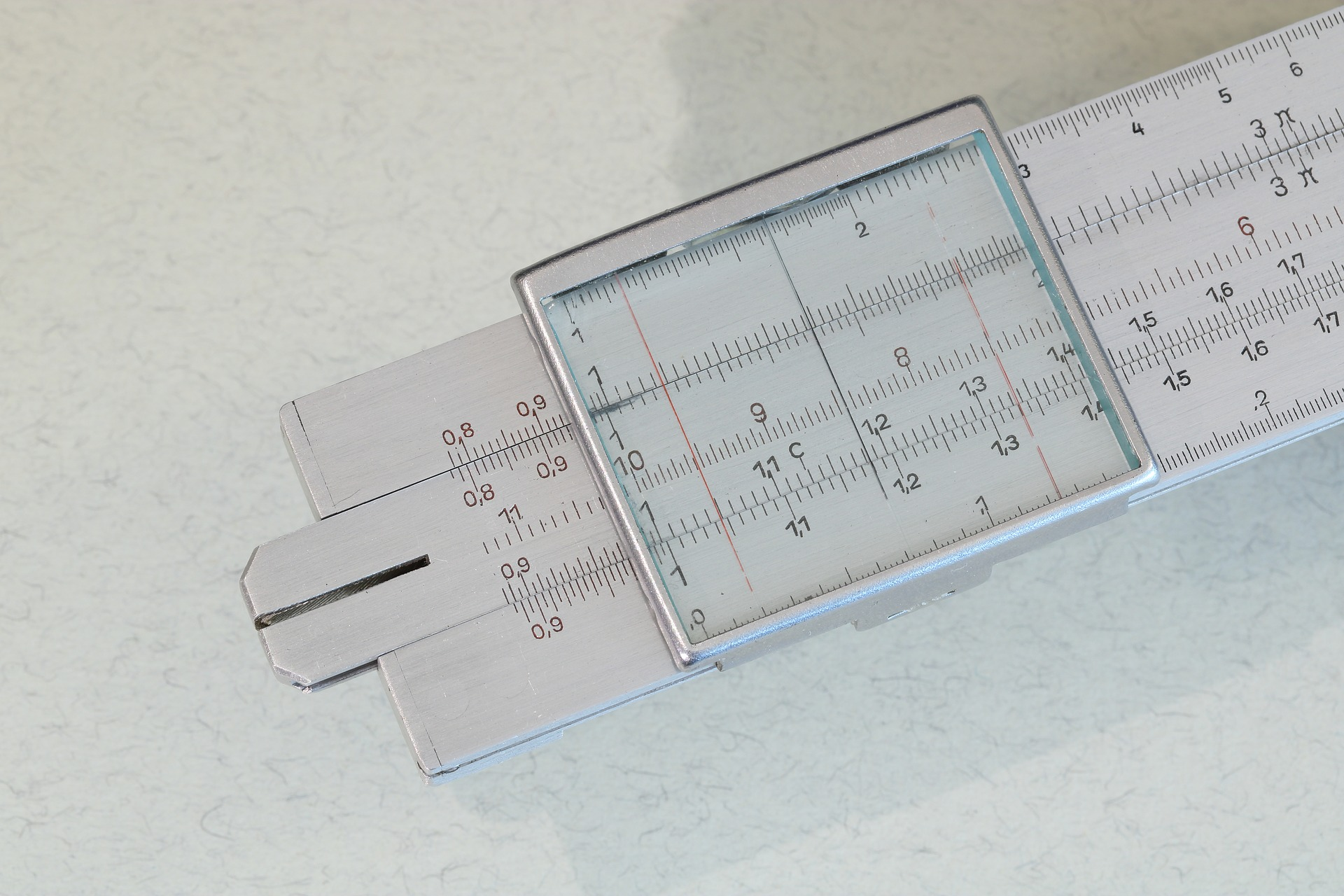Image by pixabay

Finding the measurements of a right circular cone in calculus involves practicing with formulas to find the radius, height, volume and lateral area of the cone.

When you use lateral measurements, you’ll see the terms lateral face, lateral length or lateral area of a cone mentioned in calculus problems. You may see a question prompting you to right circular cone calc: find I.

## Right Circular Cone – Basic Calculus Information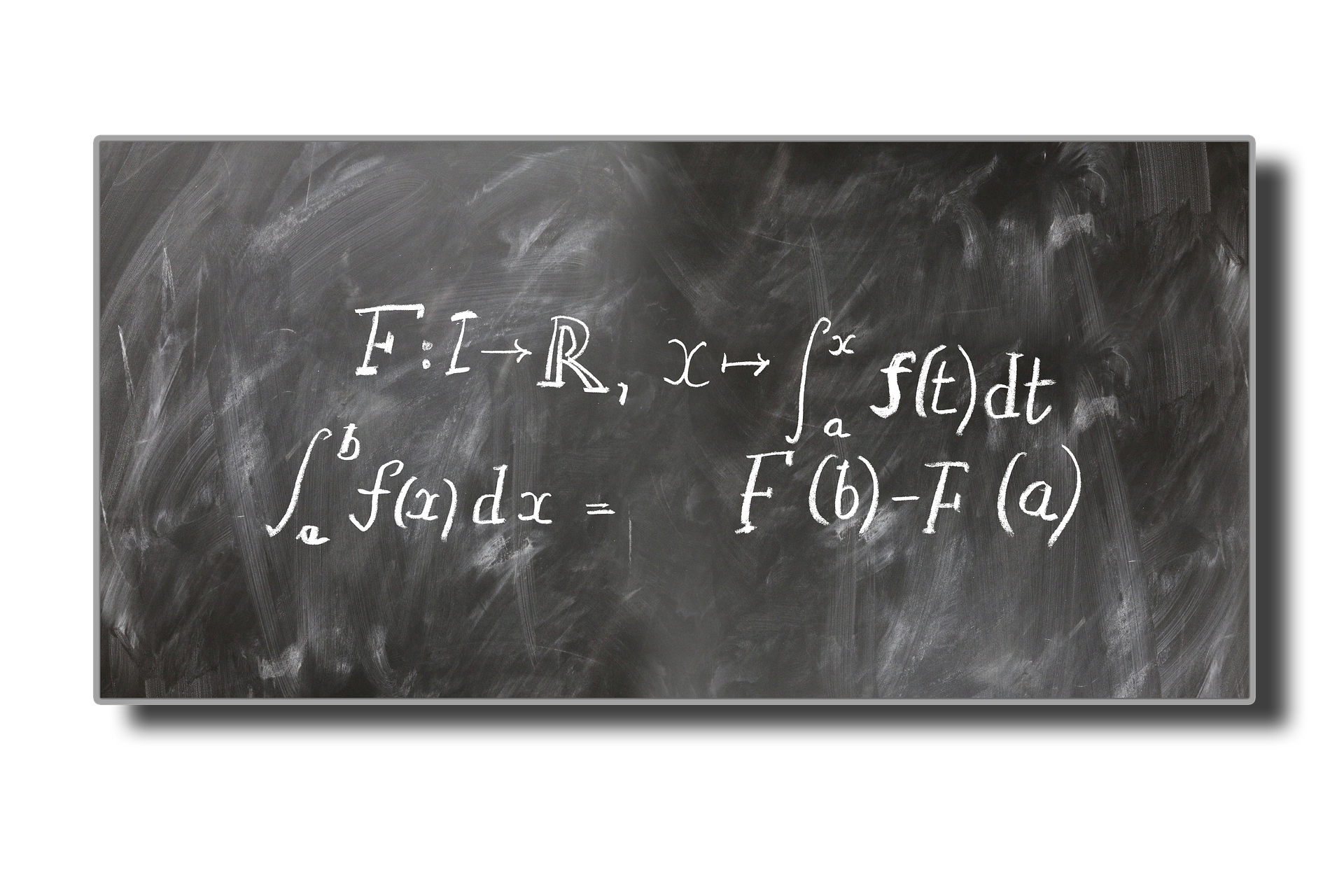Image by pixabay

A right cone has an apex directly above the center of its base, and the base isn’t necessarily a circle. A right circular cone has circular bases.

A solid geometric figure made of a circle and its base (interior) is referred to as a cone.

To solve right circular cone calc: find I, or other problems, you should learn the basics of all cones, and the meanings of the equations used to find measurements.

The cone radius equals the radius of the base. The cone altitude will be a perpendicular segment from the plane of the base to the vertex. The length of the altitude equals the height of the cone.

The cone axis consists of a segment with endpoints as the center and vertex of the base. An axis that’s perpendicular to the circle’s plane results in a right cone. When the axis isn’t perpendicular, the cone is oblique.

The slant height of a right cone is defined as the segment length from the circle of the base to the vertex of the cone. There aren’t slant height qualities for oblique cones.

Cut open a three-dimensional cone shape from the slant height, and the entire area will be the cone’s curved surface. The curved area on the cone’s surface is referred to as the lateral surface area of the cone. Therefore, the cone’s lateral surface area is the same as its curved surface area.Image by pixabay

Determine a cone’s lateral surface area with the following formula: II (Pi) times the cone radius, or R, times slant height, or SH, equal right circular cone calc: find I or L.S.A. (lateral surface area).

You’ll encounter problems describing right circular cones or cones in your studies. As long as you have the right formula, you will be able to determine the answer, but it will take some trial and error before you understand the process.

## Volume of a Cone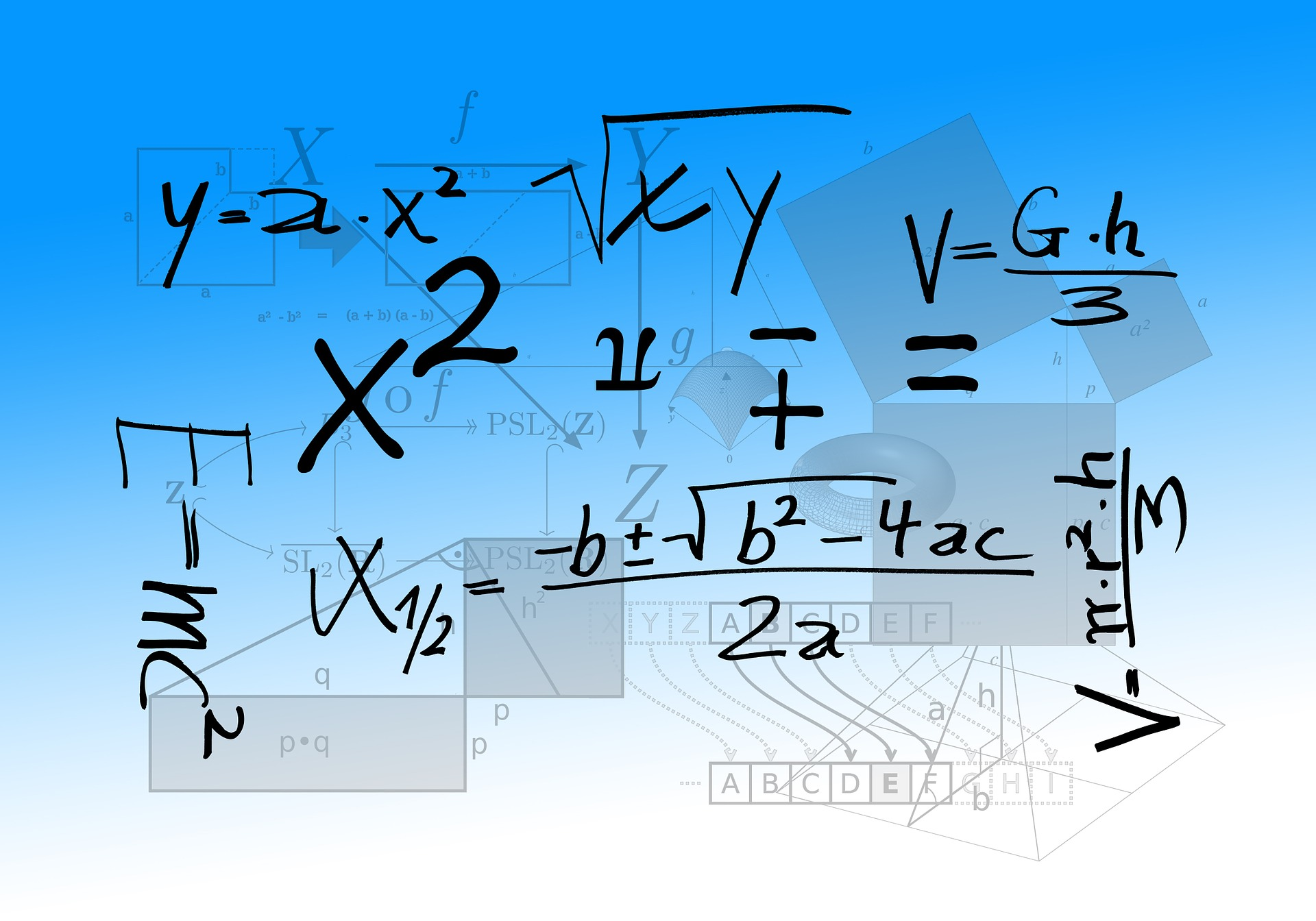Image by pixabay

A formula to figure the volume of a cone is similar to one for a pyramid. The volume (v) of a cone is 1/3 the base area, then Pi2 times the cone height. A cone has a circular base, so you need to replace the b value in a pyramid volume formula with the circle area to get the cone volume formula.

V stands for volume in cubic units, r stands for the radius in cubic units, and h equals height in units.  The equation you use will be V equals 1/3 (pi)r squared h.

## Properties to Review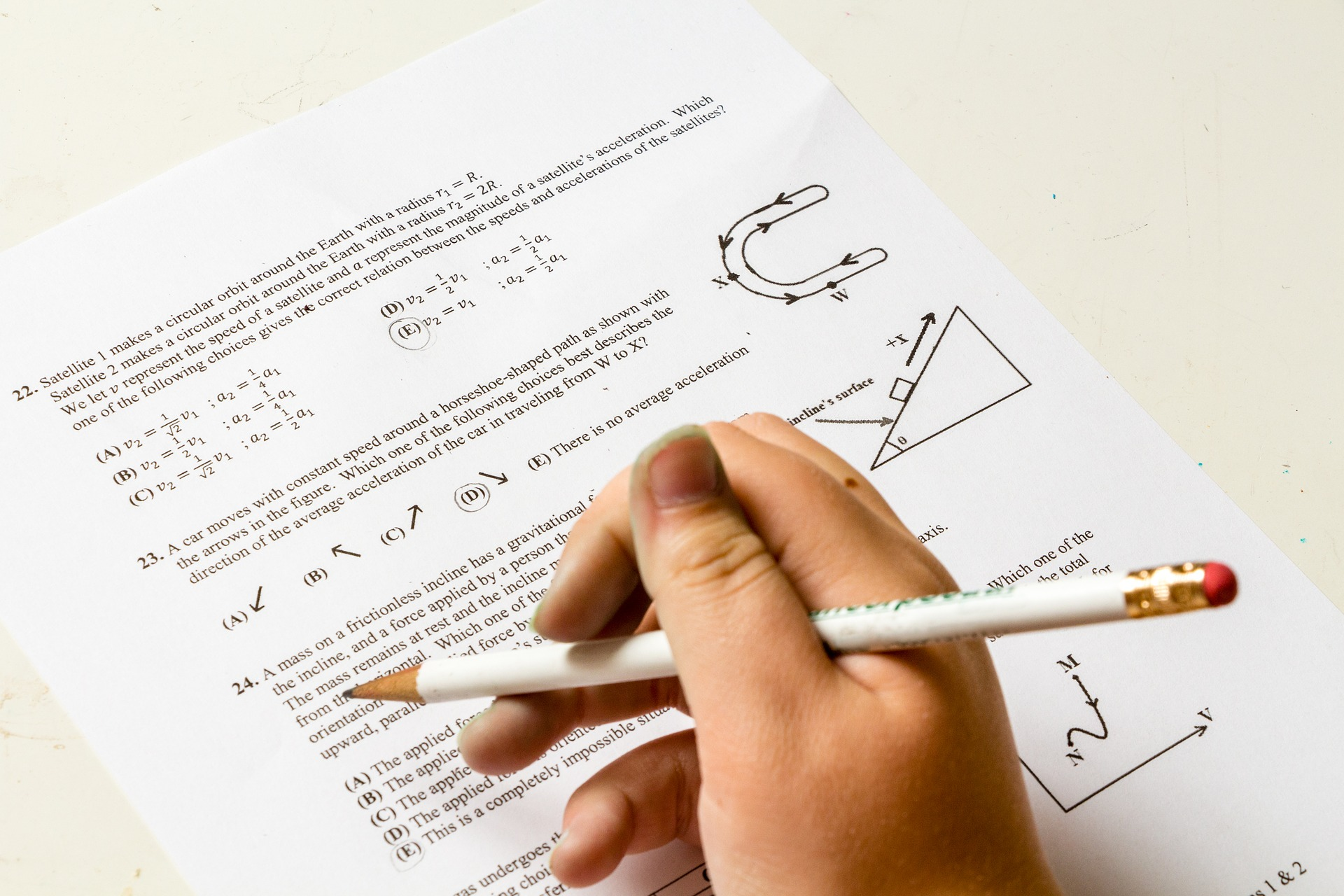Image by pixabay

Here are right circular cone properties to review:

• All parts of a right circular cone are equal

• Any part parallel to the base qualifies as a circle with a center on the cone axis.

• An isosceles triangle is defined as any part of a right circular cone that has two points of the base and the vertex.

• The length of an element is the slant height of a right circular cone.

• Elements and slant heights are usually indicated by the letter L.

A right triangle that revolves around a leg (one of its legs is the axis of the revolution) forms a right circular cone. Any surface created by the triangle hypotenuse is the right circular cone’s lateral area. The cone base is the surface created by the leg that doesn’t rotate.

## Working with Cones, Cylinders and Other Shapes

In calculus, geometry, and science, you’ll need to determine the area, volume or height of many shapes. Every shape you encounter will have a slightly different formula to help you calculate the right measurements for real-life problems or class assignments.

You can figure out the perimeter and area for 2D shapes and the volume and surface area for 3D shapes with the right formulas.  For example, calculating the area and perimeter of a triangle is easy. Add the lengths of all three sides to get the perimeter.

You’ll need to know the base and height to calculate the triangle’s area. The height is measured from the base to peak of the triangle. The formula for the triangle’s area is ½ the base times the height.

After you’ve worked with a cone, a cylinder will be easy to measure. A cylinder contains straight, parallel sides and a circular base.

You can find a cylinder’s volume or surface area if you have the height (h) and radius (r). A cylinder contains a top and a bottom, so the radius times two equals the surface area. The surface area equals 2 Pi2 plus 2pirh. The volume equals Pi2h.

The video may take a few seconds to load.

Having trouble Viewing Video content? Some browsers do not support this version – Try a different browser.## Calculating The Derivative Of Absolute Value

Calculating the derivative of absolute value is not an easy task for beginners. So our team create this post with some examples to help you how.

Calculating the derivative of absolute value is challenging at first, but once you learn the formula, you can easily find the right values and functions in any problem. You will need to use many terms when working with derivatives, including continuity, discontinuity, piecewise, limits, and differential.

## How To Calculate The Derivative of Absolute Value

Derivatives are functions of a single variable at a certain value, and a derivative represents the slope of the tangent line about the function graph at the chosen point. Derivatives represent a basic tool used in calculus.

A derivative will measure the depth of the graph of a function at a random point on the graph. Therefore, the chosen derivative is called a slope. The derivative has a ratio of change in the function value to adjustment in the free variable.

Learn about derivatives, limits, continuity, and other components so you can calculate the derivative of absolute value in mathematics.

## Overview of Derivatives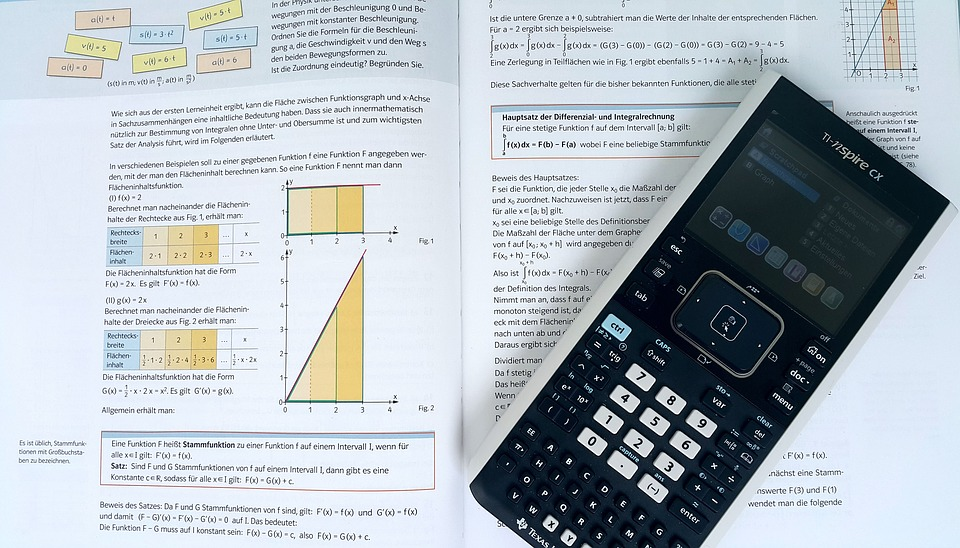Image by Pixabay

You need to know the value of the function or f(x) at a certain x, but you may also want to find out how fast the function changes. The slope of the line indicates the linear function. The slope is different at every point for a curved line. A constant number shows the slope of a straight line, and a curved line slope is a function of x.

Use the derivative or f prime of x, which is written as f(x) to find the value of lines. You’ll find many curved lines in calculus. Read all you can about limits and practice using them since they are essential when you work with derivatives.

Derivatives are sometimes used to find out a rate of change, but they can also approximate local non-linear functions. A real variable or real-valued function and a surface defined by a multi-variable function may use derivatives.

A linear function is seen as the line tangent at a particular point and can be seen in a real variable as a tangent plane. A derivative may be the best linear representation for a function in the area of a point.

Simply put, derivatives represent how a quantity changes while another quantity varies. You can make models by comparing quantities to their rates of change, Use tools from differential equations to predict the rate of change. The predictions made using derivatives are usually accurate.

When you work with derivatives, you’ll also need to know the following terms:

• Continuity

• Discontinuity

• Limits

• Differential

• Square root

• Piecewise

Some of these terms are explained below and in the derivate examples.

## Limits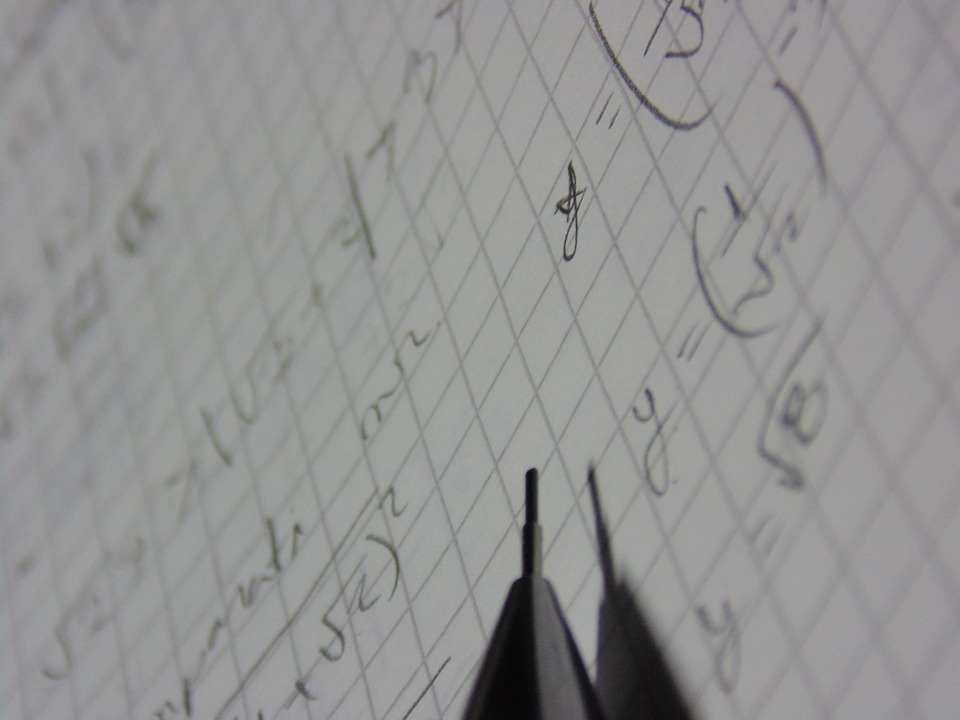Image by Pixabay

Here’s one example of limits and how to use them. Look at the function f(x)=x+2f(x)=x+2. Study the graphed function to find the limit of ff is x equals 3x equals 3 pinpoints the value of ff when you get close to x equals 3x equals 3.

The yy value you get close to when you look at the ff graph and come closer to the x equals 3s equals 3 point as the limit. If you begin at the (1,3) and (1,3) point on a graph and move until you get next to x equals 3x equals 3, then the function’s value, or yy value, is almost at 55.

When you begin at (5,&0 and (5,7) on the graph, move to the left until you’re almost at x equals 3x equals 3. you’ll notice the yy value will be almost 55 again. The limit of ff, then, is x equals 3x equals 3 equals 55.

## Continuous and Discontinuous FunctionsImage by Pixabay

A continuous function has no points missing or jumps – the graph is linked everywhere you look.

A single-step discontinuity function can be exampled as follows:

A function with zero applied to x values less than zero, and one for x values equal to or greater than zero has a single-step discontinuity at the x equals zero point. In a graph of this discontinuous function, there’s an open circle, or (0,0), which indicates one point is absent from the function.

The graph f(x), however, includes point (0,1) as a filled in circle; therefore, it is a simple discontinuous function.

The unit-step function has a step at zero. When x gets close to zero from below, the result is different than the limit when x gets close to zero from a higher point on the graph.

Check a graph drawn to show this unit step function, and you’ll find that x equals zero is discontinuous. You’ll see that f(x) is continuous elsewhere on the graph. One discontinuity causes the function to be discontinuous to its real number domain.

A jump discontinuity can also cause a function to become discontinuous. A function can be zero in all places on the graph except x equals zero, a location where it becomes one. The discontinuity is called a jump discontinuity.

You’ll find an open circle at the (0,0) point to show the point is not on the graph. A closed circle (0,1) shows that the point is included. The equation for this jump discontinuity reads  0 to the zero power equals 0, lim f(x) equals zero and a second lim f(x) equals zero.

This particular function has the same right and left limits. Then, we compare the limit and function value, which reads lim f(x) equals zero and (f) 0 equals 1. The values aren’t the same and are discontinuous.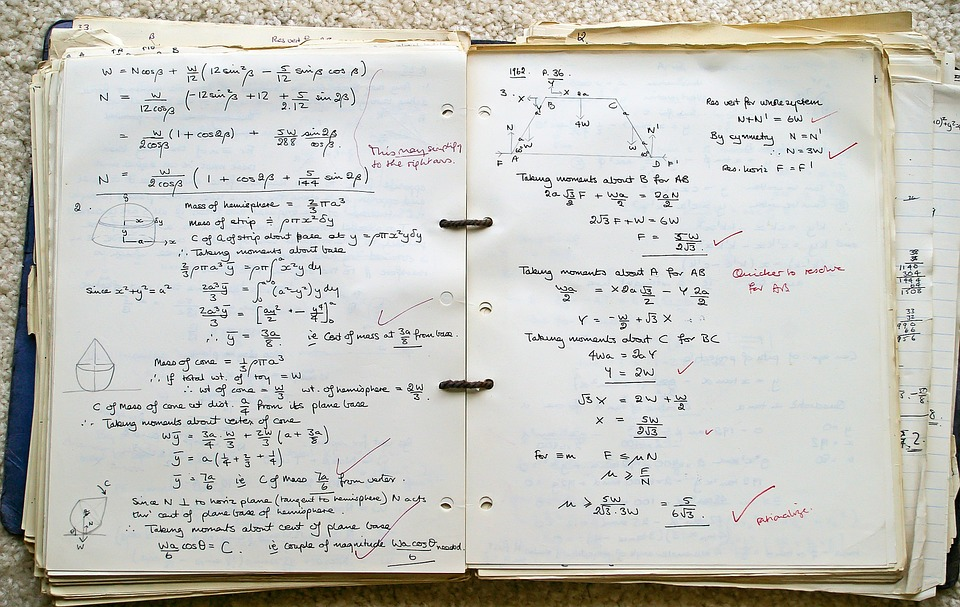Image by Pixabay

One statement about continuity says that the limit of the function right before x gets to x0 from the left is the same as when x gets close to x0 from the right. Both the right and left limits are the same; you can call them as the limit of f(x) when x gets close to x0. When the left and right limits are the same at a certain point, there are limits at the point.

Another statement about continuity says that the limit should be equal to the function value at the determined point. When the function fails the right and left limit matching scenario, it isn’t continuous at zero and fails the continuity test.

## Summary of How to Find the Derivative of Absolute Value

If you plot the graph of |x|, you’ll see that there are only two possible slopes, which are +1 when x is positive and -1 when x is negative. Also, you cannot define this slope when x is 0.

So, a simple way of showing this is in an expression as |x|/x, which turns out to be 1 when x is positive and -1 when x is negative.

Finally, the derivative of absolute value is abs(x)/x.

Do you have any additional tips to finding the absolute value of x? Let us know in a comment!

The video may take a few seconds to load.Having trouble Viewing Video content? Some browsers do not support this version – Try a different browser.